﻿• 价格透明
• 信息保密
• 进度掌控
• 售后无忧# elegant-visualization-of-density-distribution-in-r-using-ridgeline

Basic density ridgeline plots Key R functions:

geom_density_ridges(): Estimates data densities and then draws those
using ridgelines. It arranges multiple density plots in a staggered
fashion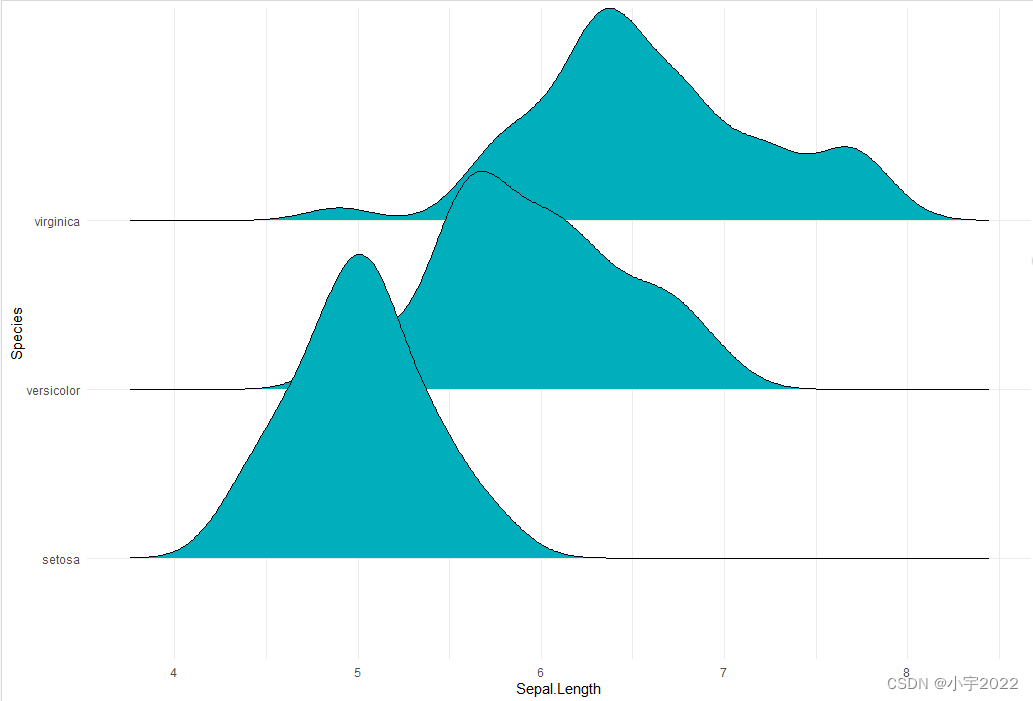``````library(ggplot2)
library(ggridges)
theme_set(theme_minimal())
# Opened polygons
ggplot(iris, aes(x = Sepal.Length, y = Species, group = Species)) +
geom_density_ridges(fill = "#00AFBB")
``````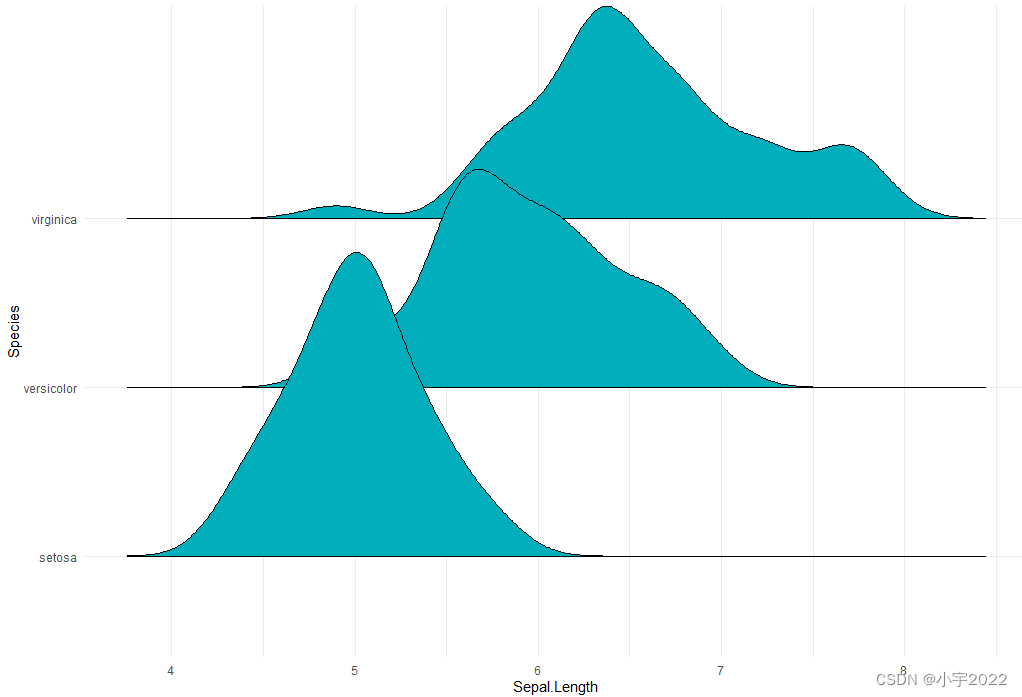``````# Closed polygons
ggplot(iris, aes(x = Sepal.Length, y = Species, group = Species)) +
geom_density_ridges2(fill = "#00AFBB")
``````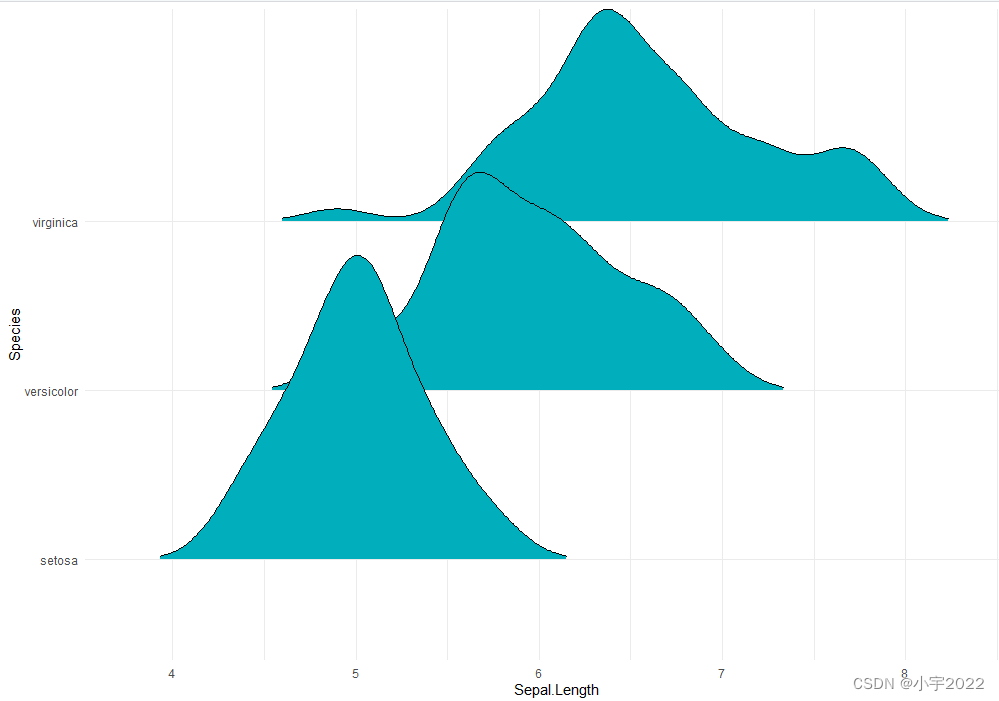``````# Cut off the trailin tails.
# Specify `rel_min_height`: a percent cutoff
ggplot(iris, aes(x = Sepal.Length, y = Species)) +
geom_density_ridges(fill = "#00AFBB", rel_min_height = 0.01)
``````

Note that, the grouping aesthetic does not need to be provided if a
categorical variable is mapped onto the y axis, but it does need to be
provided if the variable is numerical.

Control the extent to which the different densities overlap. You can
control the overlap between the different density plots using the
scale option. Default value is 1. Smaller values create a separation
between the curves, and larger values create more overlap.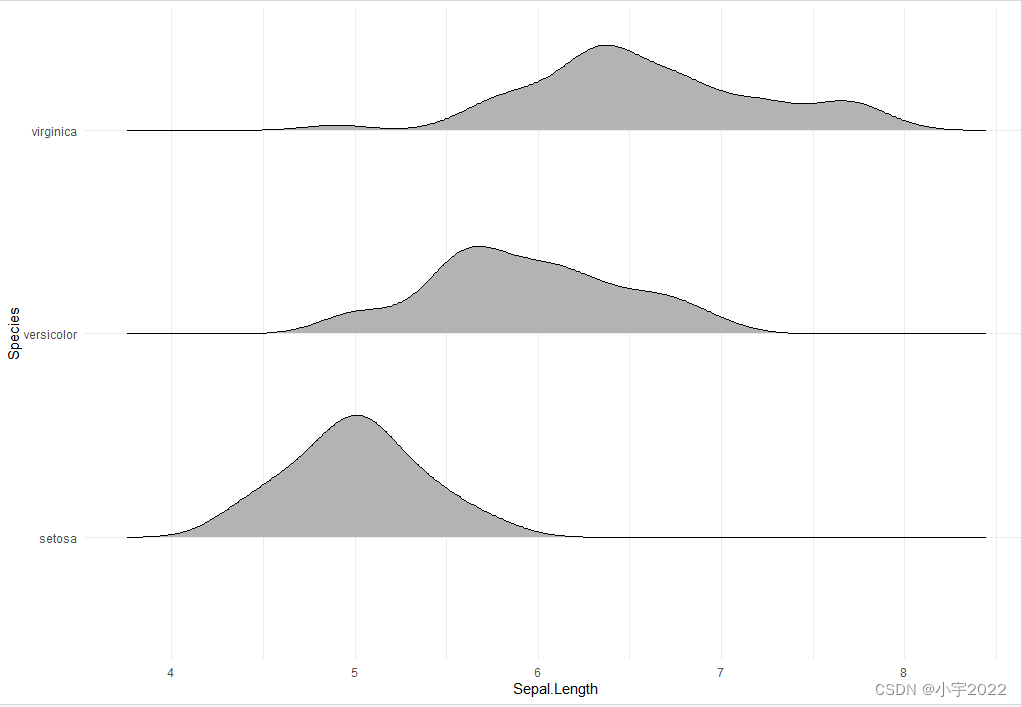``````library(ggplot2)
library(ggridges)
theme_set(theme_minimal())
# scale = 0.6, not touching
ggplot(iris, aes(x = Sepal.Length, y = Species)) +
geom_density_ridges(scale = 0.6)
``````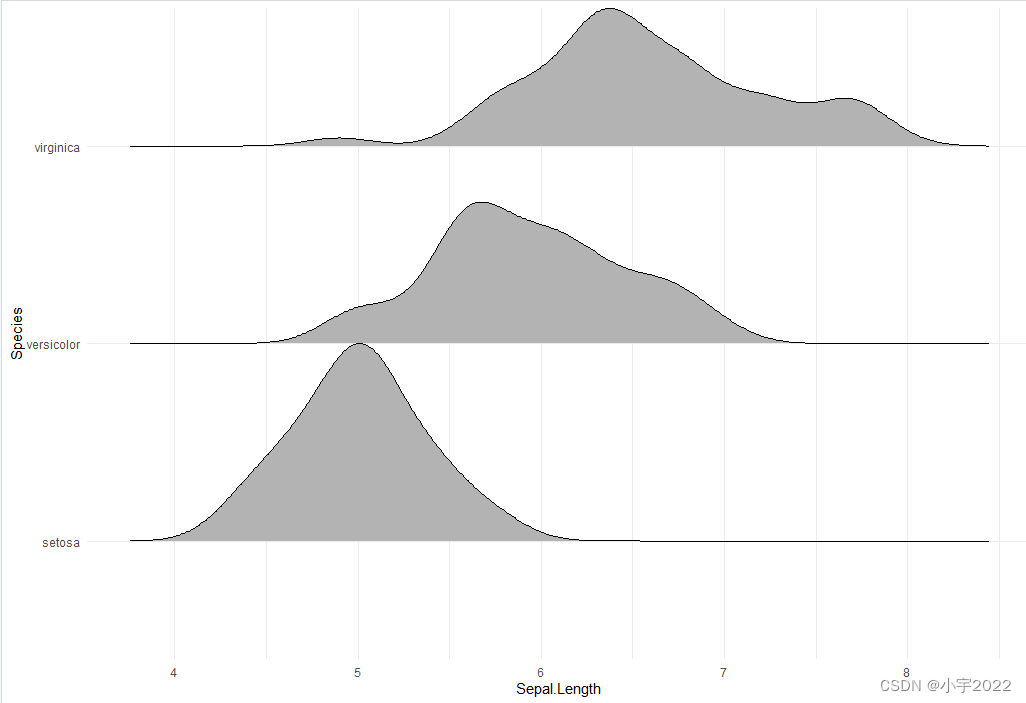``````# scale = 1, exactly touching
ggplot(iris, aes(x = Sepal.Length, y = Species)) +
geom_density_ridges(scale = 1)
``````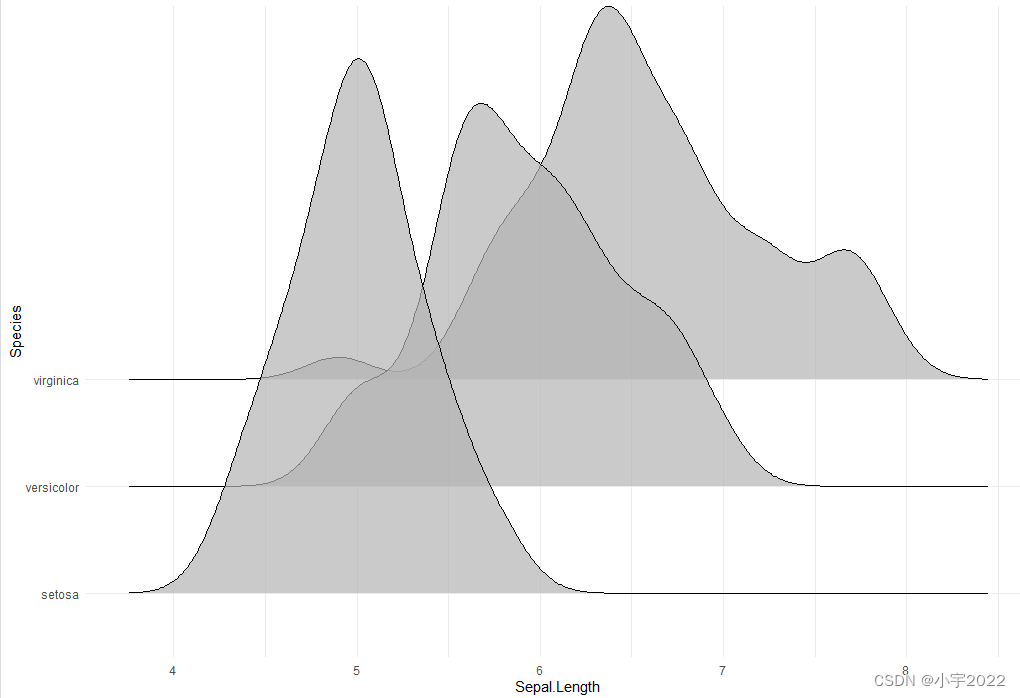``````# scale = 5, substantial overlap
ggplot(iris, aes(x = Sepal.Length, y = Species)) +
geom_density_ridges(scale = 5, alpha = 0.7)
``````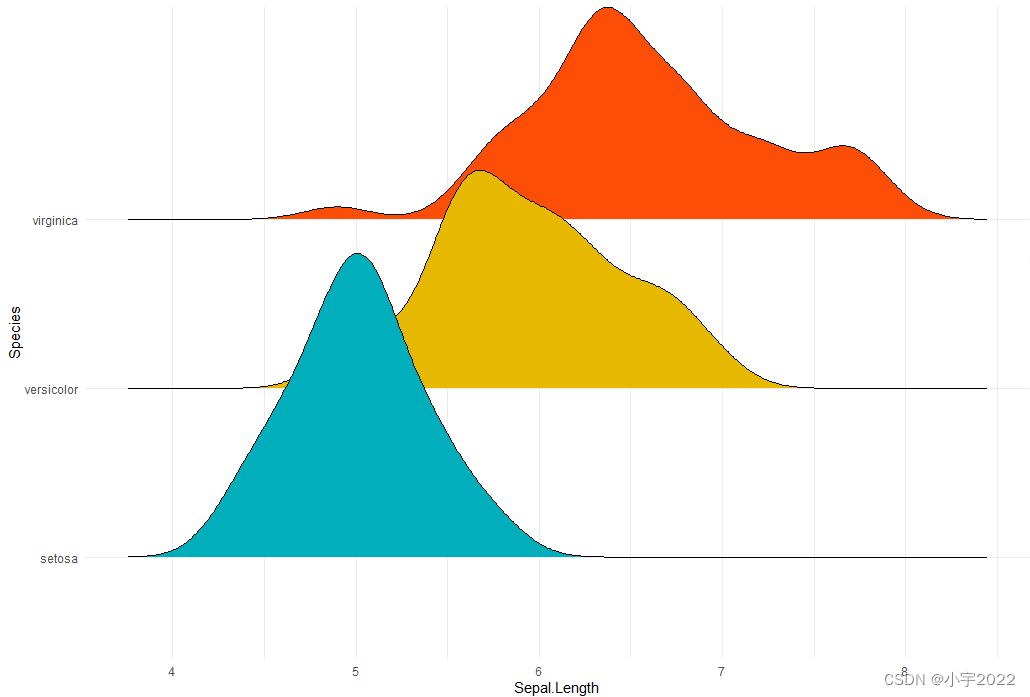``````ggplot(iris, aes(x = Sepal.Length, y = Species)) +
geom_density_ridges(aes(fill = Species)) +
scale_fill_manual(values = c("#00AFBB", "#E7B800", "#FC4E07")) +
theme(legend.position = "none")
``````

Density curves with gradient fill colors along the x axis This effect
can be achieved with the function geom_density_ridges_gradient(),
which works like geom_density_ridges, except that it allows for
varying fill colors.

Note that, for technical reasons, the geom_density_ridges_gradient()
do not allow for alpha transparency in the fill.

Visualize the Lincoln weather data:

Data set: lincoln_weather [in ggridges]. Weather in Lincoln, Nebraska
in 2016. Create the density ridge plots of the Mean Temperature by
Month and change the fill color according to the temperature value (on
x axis).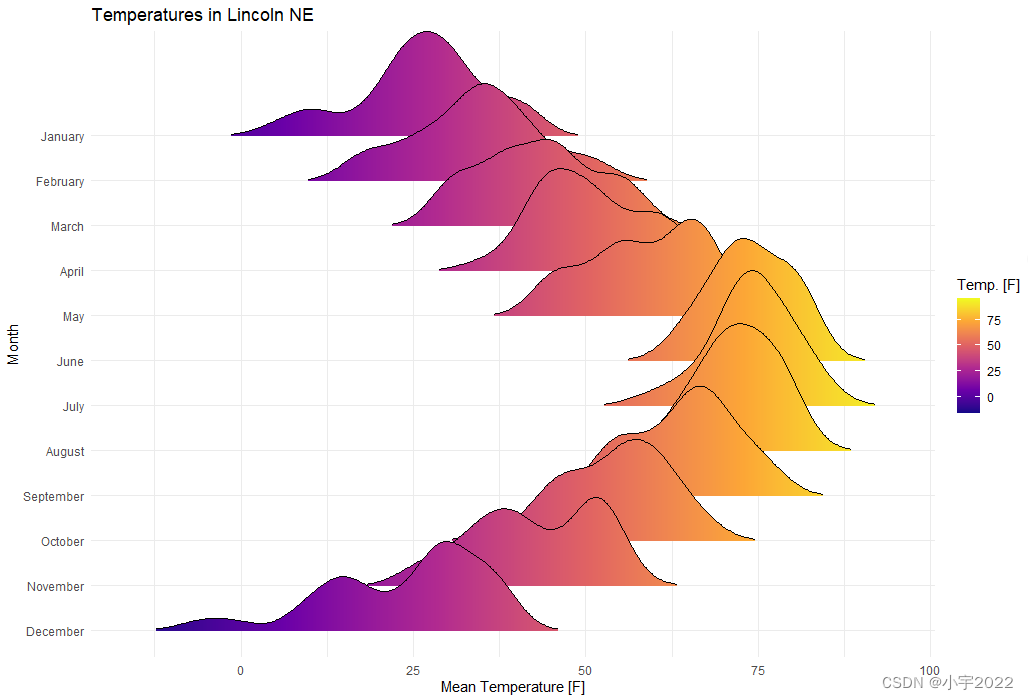``````library(ggplot2)
library(ggridges)
theme_set(theme_minimal())
# Change the density area fill colors by groups
ggplot(
lincoln_weather,
aes(x = `Mean Temperature [F]`, y = `Month`, fill = stat(x))
) +
geom_density_ridges_gradient(scale = 3, size = 0.3, rel_min_height = 0.01) +
scale_fill_viridis_c(name = "Temp. [F]", option = "C") +
labs(title = 'Temperatures in Lincoln NE')
``````

stat_density_ridges(). By default, three lines are drawn,
corresponding to the first, second, and third quartile.``````library(ggplot2)
library(ggridges)
theme_set(theme_minimal())
# Add quantiles Q1, Q2 (median) and Q3
ggplot(iris, aes(x = Sepal.Length, y = Species)) +
stat_density_ridges(quantile_lines = TRUE)
``````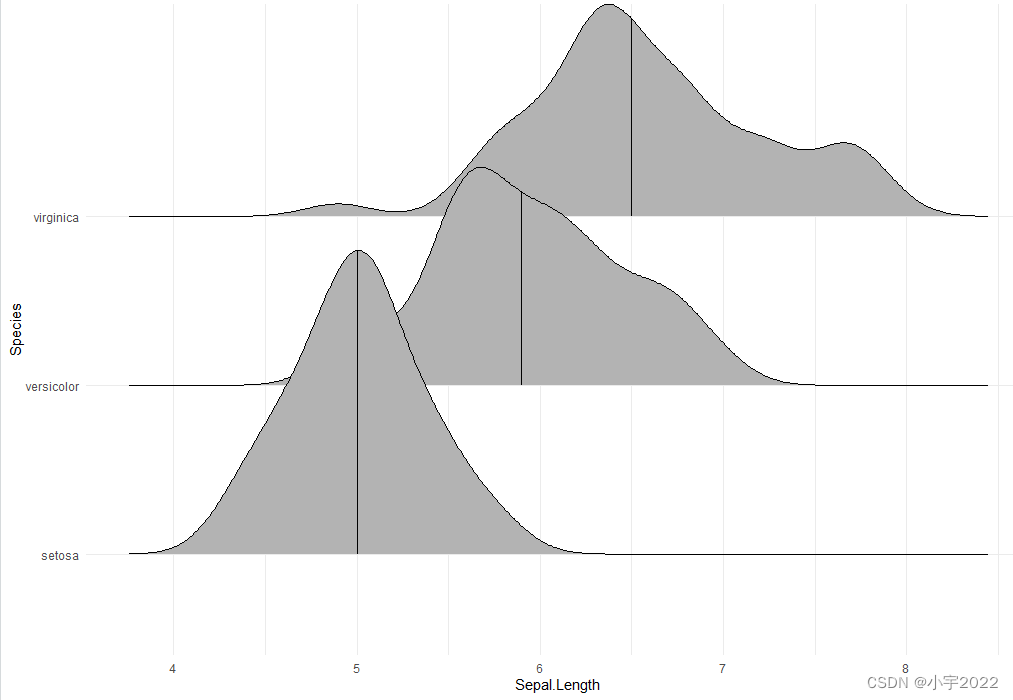``````# Show only the median line (50%)
# Use quantiles = 2 (for Q2) or quantiles = 50/100
ggplot(iris, aes(x = Sepal.Length, y = Species)) +
stat_density_ridges(quantile_lines = TRUE, quantiles = 0.5)
``````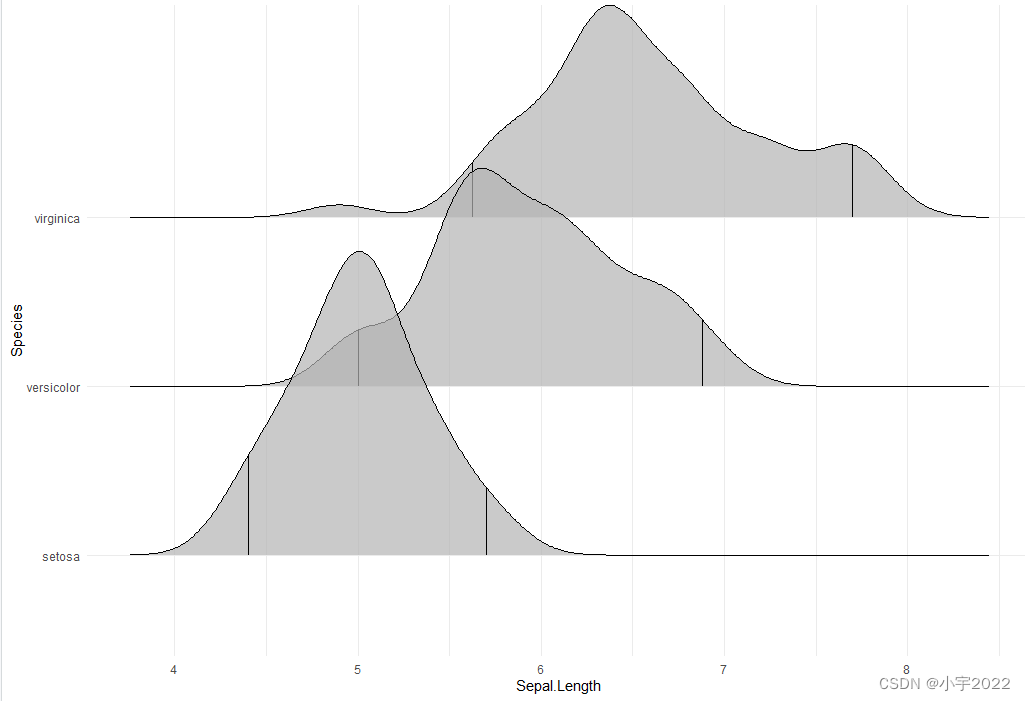``````# Indicate the 2.5% and 97.5% tails
ggplot(iris, aes(x = Sepal.Length, y = Species)) +
stat_density_ridges(quantile_lines = TRUE, quantiles = c(0.025, 0.975), alpha = 0.7)
``````

Color the density area by quantiles You need to specify the option
calc_ecdf = TRUE required for calculating quantiles. The ECDF
represents the empirical cumulative density function for the
distribution.

``````library(ggplot2)
library(ggridges)
theme_set(theme_minimal())
# Color by quantiles
ggplot(iris, aes(x = Sepal.Length, y = Species, fill = factor(stat(quantile)))) +
stat_density_ridges(
geom = "density_ridges_gradient", calc_ecdf = TRUE,
quantiles = 4, quantile_lines = TRUE
) +
scale_fill_viridis_d(name = "Quartiles")
``````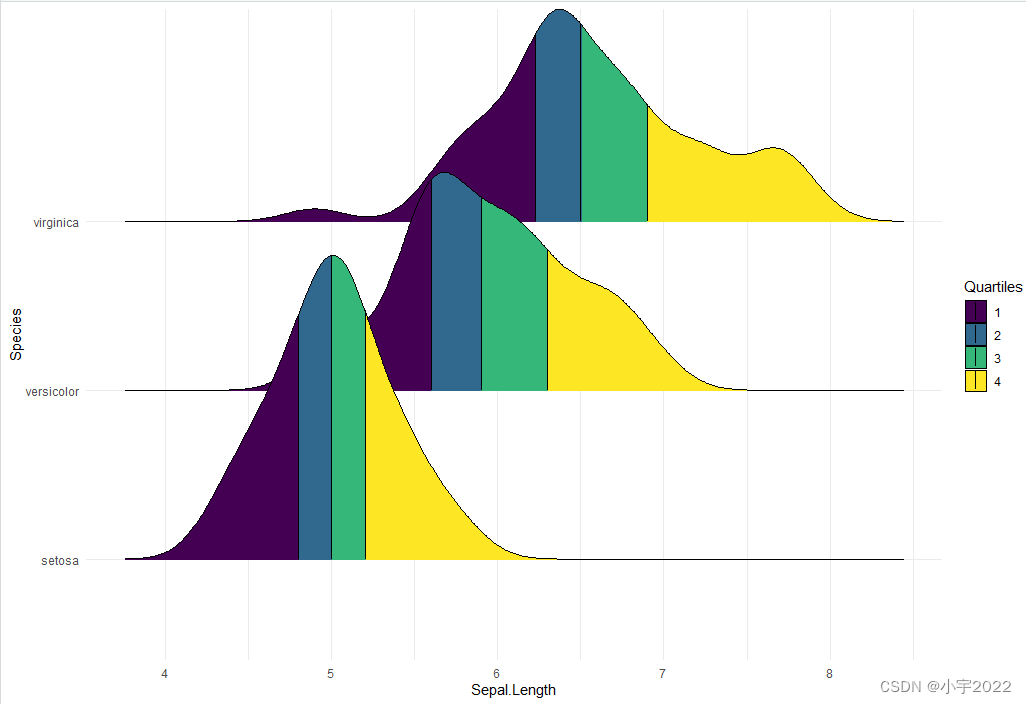``````# Highlight the tails of the distributions
ggplot(iris, aes(x = Sepal.Length, y = Species, fill = factor(stat(quantile)))) +
stat_density_ridges(
calc_ecdf = TRUE,
quantiles = c(0.025, 0.975)
) +
scale_fill_manual(
name = "Probability", values = c("#FF0000A0", "#A0A0A0A0", "#0000FFA0"),
labels = c("(0, 0.025]", "(0.025, 0.975]", "(0.975, 1]")
)
``````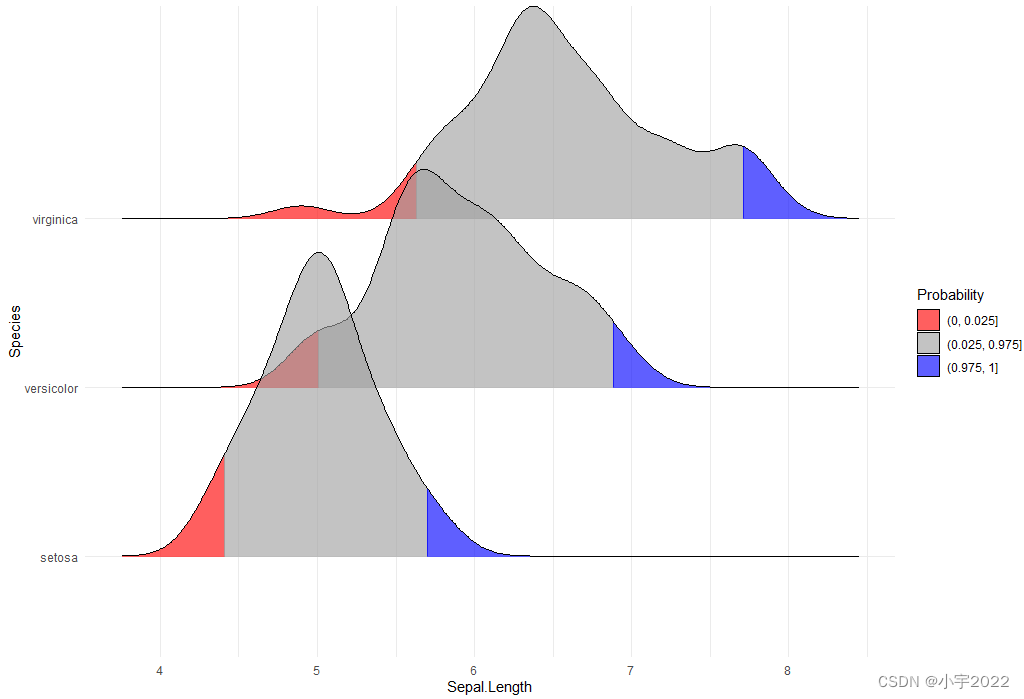Color the density curves by probabilities When calc_ecdf = TRUE, we
represents the empirical cumulative density function for the
distribution. This allows us to map the probabilities directly onto
color.

``````ggplot(iris, aes(x = Sepal.Length, y = Species, fill = 0.5 - abs(0.5 - stat(ecdf)))) +
stat_density_ridges(geom = "density_ridges_gradient", calc_ecdf = TRUE) +
scale_fill_viridis_c(name = "Tail probability", direction = -1)
``````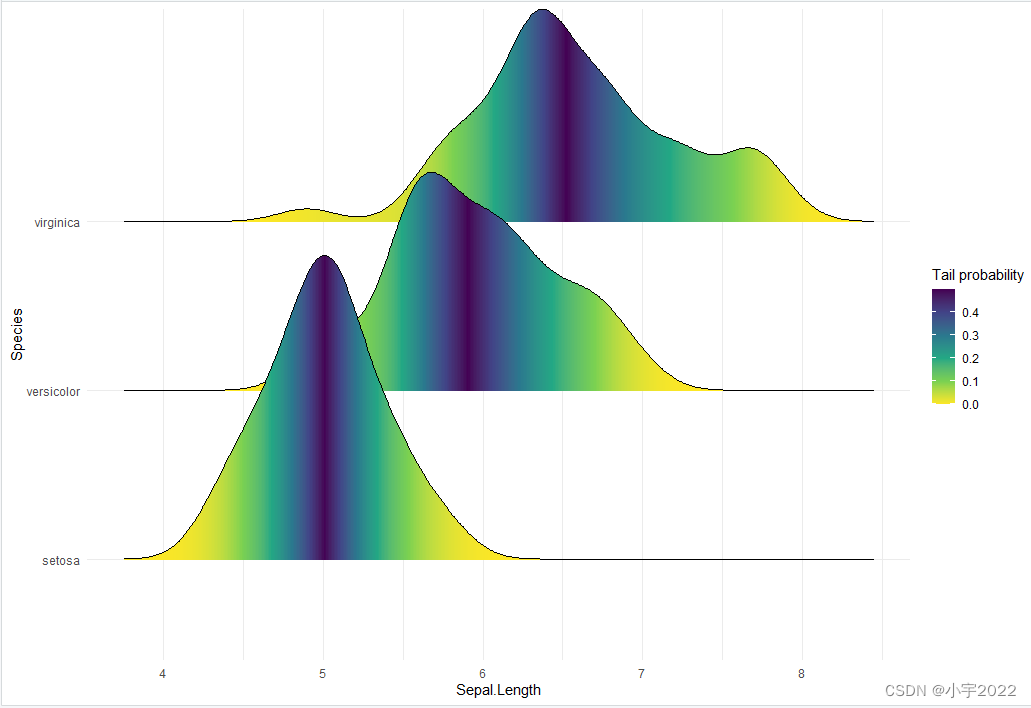Add the original data points onto the density curves This can be done
by setting jittered_points = TRUE, either in stat_density_ridges or in
geom_density_ridges.

The position of data points can be controlled using the following
options:

position = “sina”: Randomly distributes points in a ridgeline plot
between baseline and ridgeline. This is the default option. position =
“jitter”: Randomly jitter the points in a ridgeline plot. Points are
randomly shifted up and down and/or left and right. position =
“raincloud”: Creates a cloud of randomly jittered points below a
ridgeline plot.

``````# Add jittered points
ggplot(iris, aes(x = Sepal.Length, y = Species)) +
geom_density_ridges(jittered_points = TRUE)
``````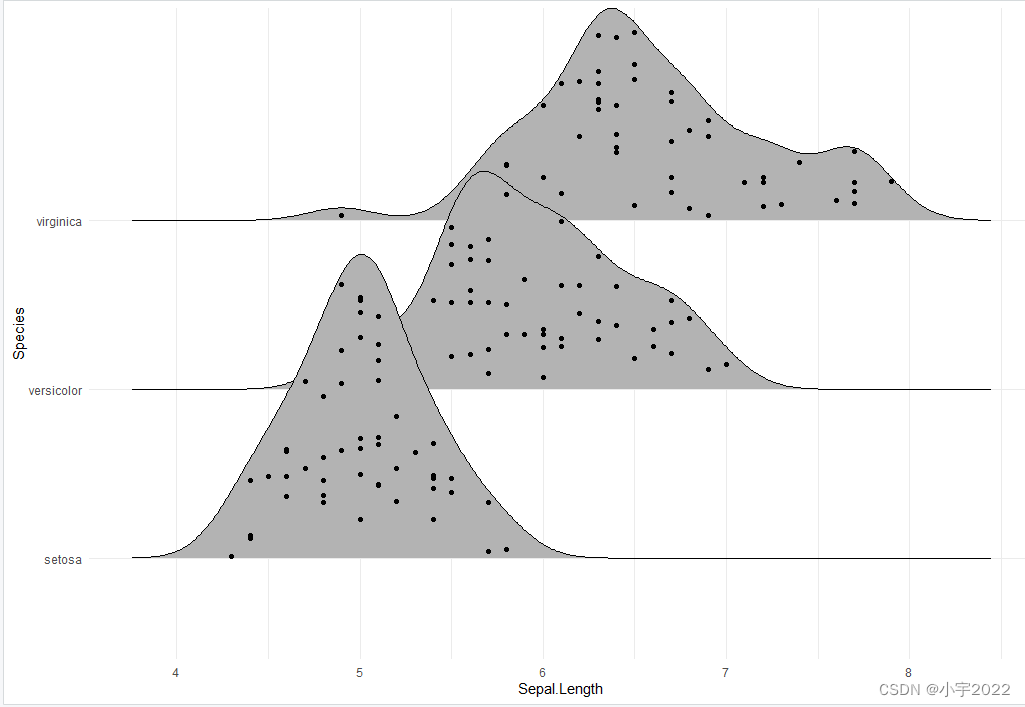``````# Control the position of points
# position = "raincloud"
ggplot(iris, aes(x = Sepal.Length, y = Species)) +
geom_density_ridges(
jittered_points = TRUE, position = "raincloud",
alpha = 0.7, scale = 0.9
)
``````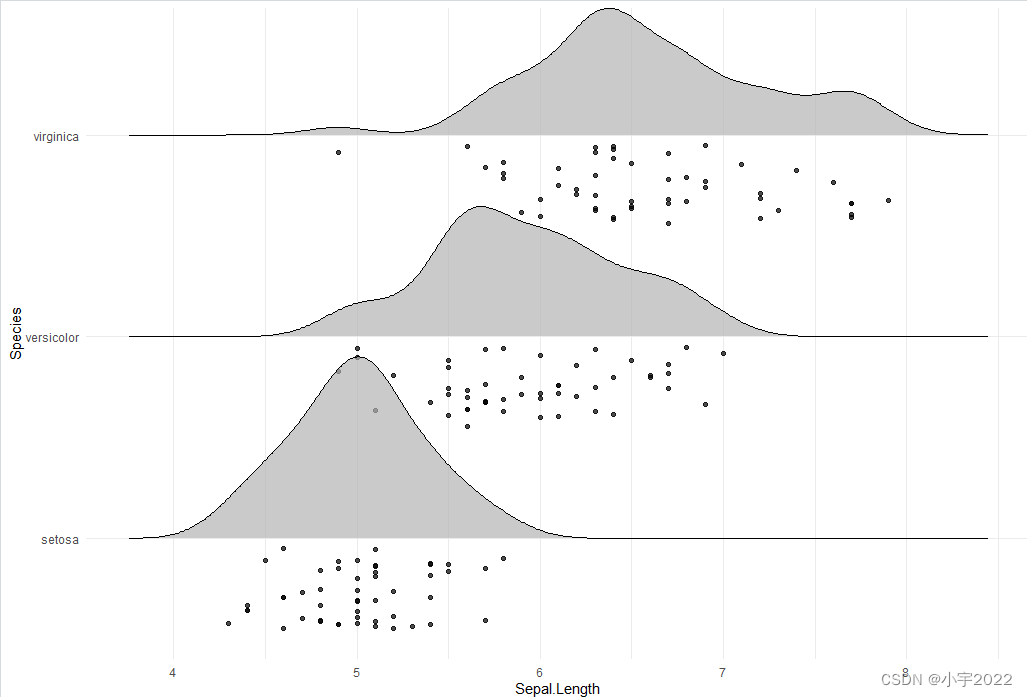``````# position = "points_jitter"
ggplot(iris, aes(x = Sepal.Length, y = Species)) +
geom_density_ridges(
jittered_points = TRUE, position = "points_jitter",
alpha = 0.7, scale = 0.9
)
``````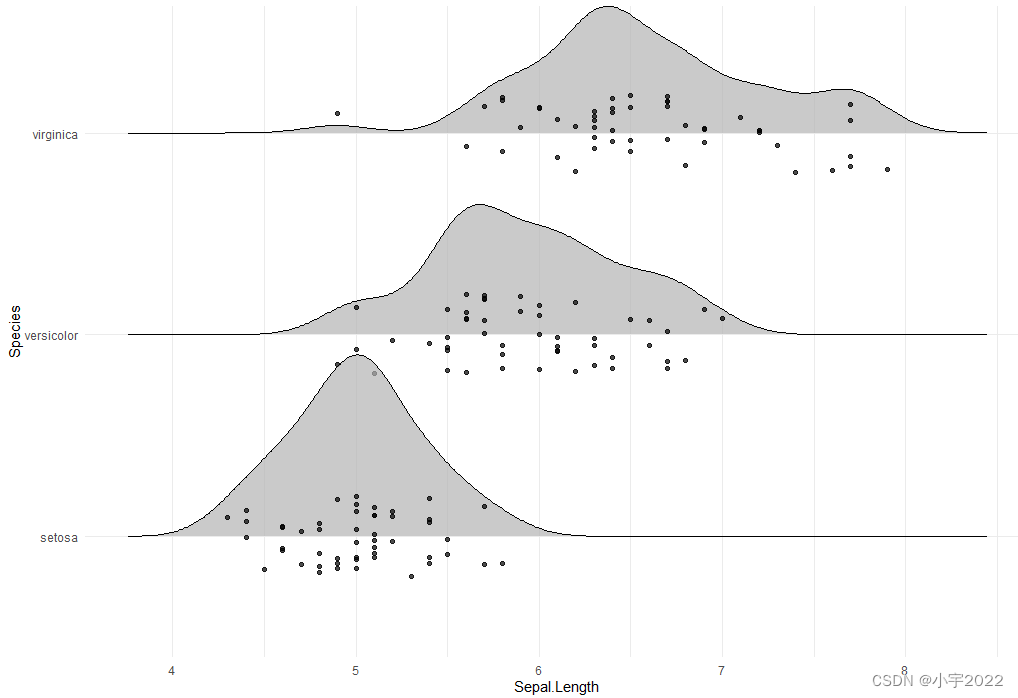``````# Add marginal rug
ggplot(iris, aes(x = Sepal.Length, y = Species)) +
geom_density_ridges(
jittered_points = TRUE,
position = position_points_jitter(width = 0.05, height = 0),
point_shape = '|', point_size = 3, point_alpha = 1, alpha = 0.7,
)
``````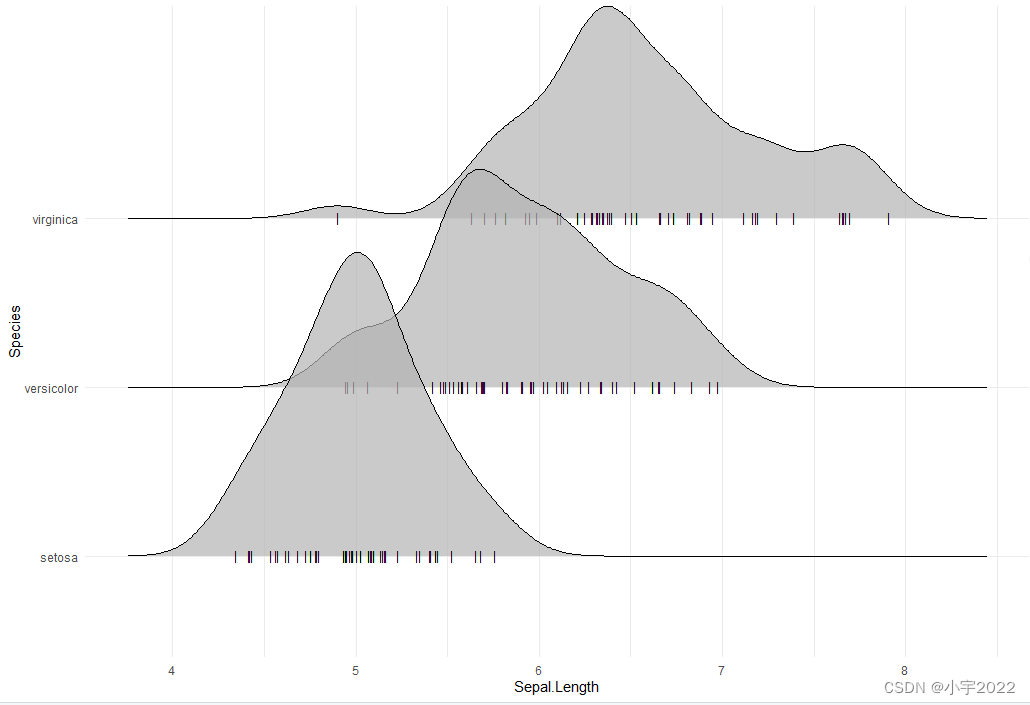Styling the jittered points and add quantile lines.

``````# Styling jittered points
ggplot(iris, aes(x = Sepal.Length, y = Species, fill = Species)) +
geom_density_ridges(
aes(point_color = Species, point_fill = Species, point_shape = Species),
alpha = .2, point_alpha = 1, jittered_points = TRUE
) +
scale_point_color_hue(l = 40) +
scale_discrete_manual(aesthetics = "point_shape", values = c(21, 22, 23))
``````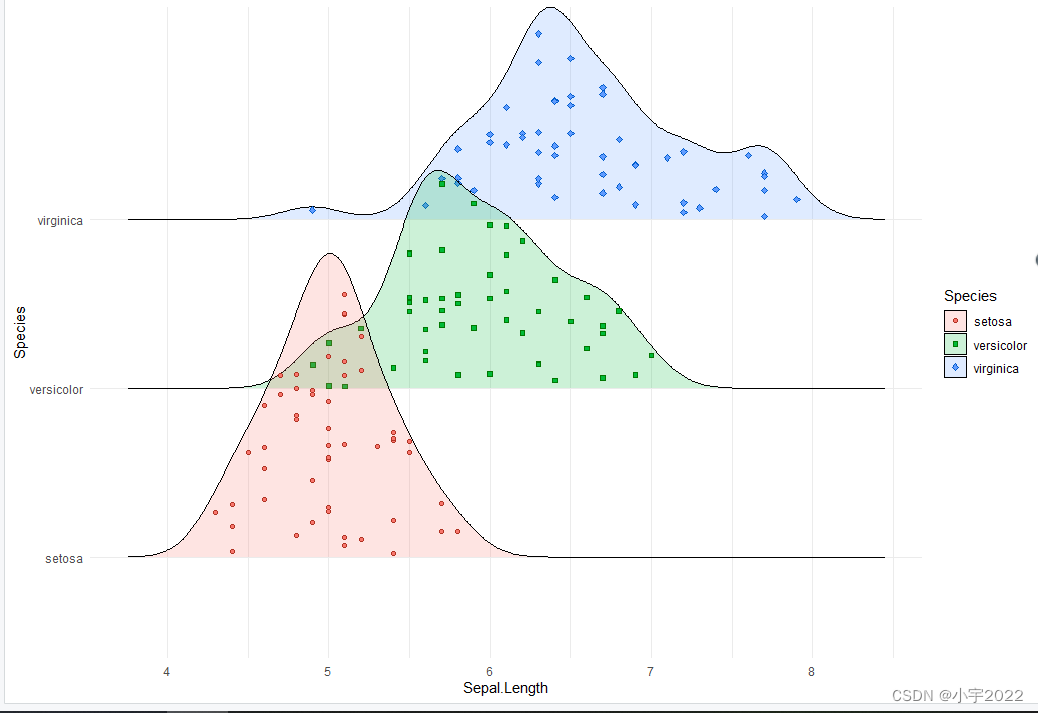``````# Styling vertical quantile lines
ggplot(iris, aes(x = Sepal.Length, y = Species)) +
geom_density_ridges(
jittered_points = TRUE, quantile_lines = TRUE, scale = 0.9, alpha = 0.7,
vline_size = 1, vline_color = "red",
point_size = 0.4, point_alpha = 1,
)
``````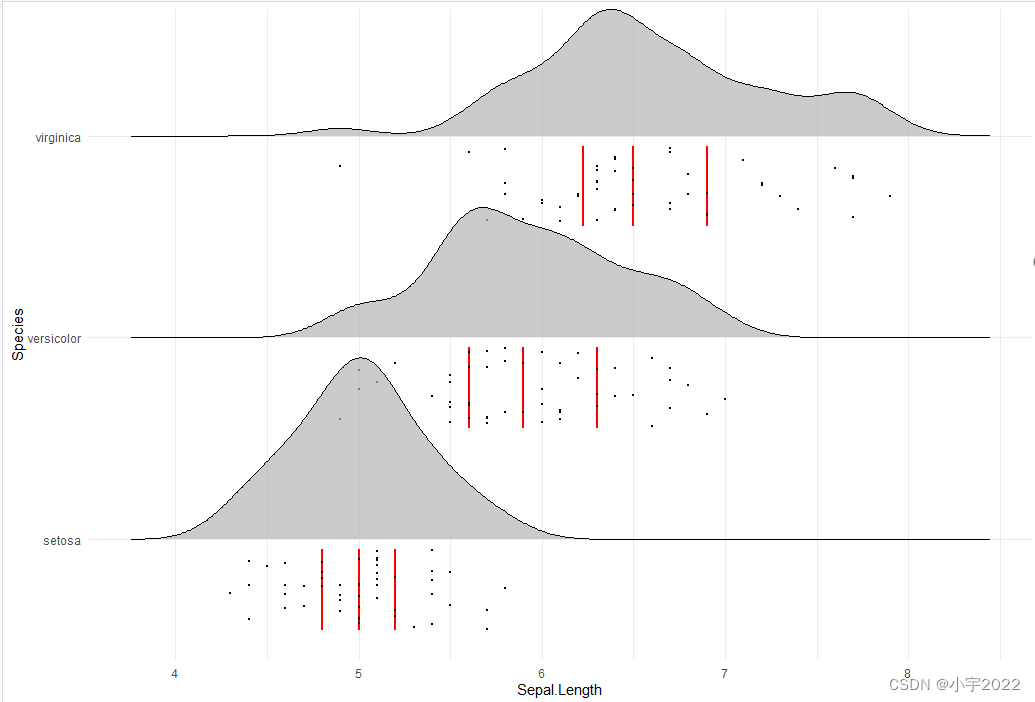Create histogram distributions using ridgeline Use the following
options:

stat = “binline”: Creates histogram plots draw_baseline = FALSE:
Removes trailing lines to either side of the histogram. For
histograms, the rel_min_height parameter doesn’t work very well.

``````ggplot(iris, aes(x = Sepal.Length, y = Species, height = stat(density))) +
geom_density_ridges(
stat = "binline", bins = 20, scale = 0.95,
draw_baseline = FALSE
)
``````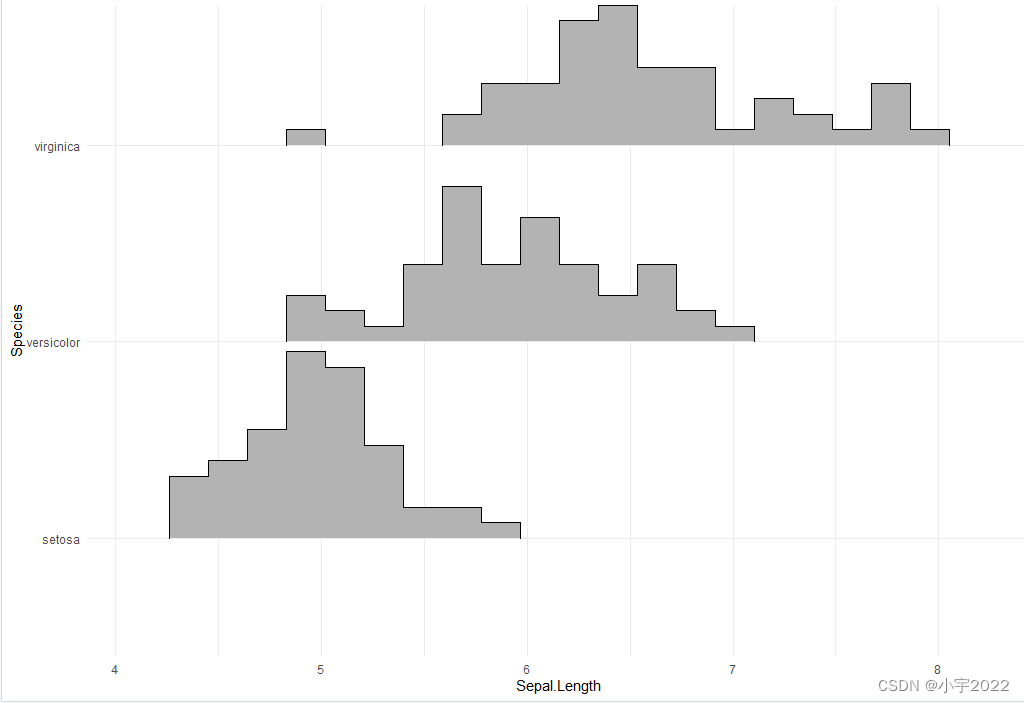Change themes

``````# Use theme_ridges()
ggplot(iris, aes(x = Sepal.Length, y = Species)) +
geom_density_ridges() +
theme_ridges()
``````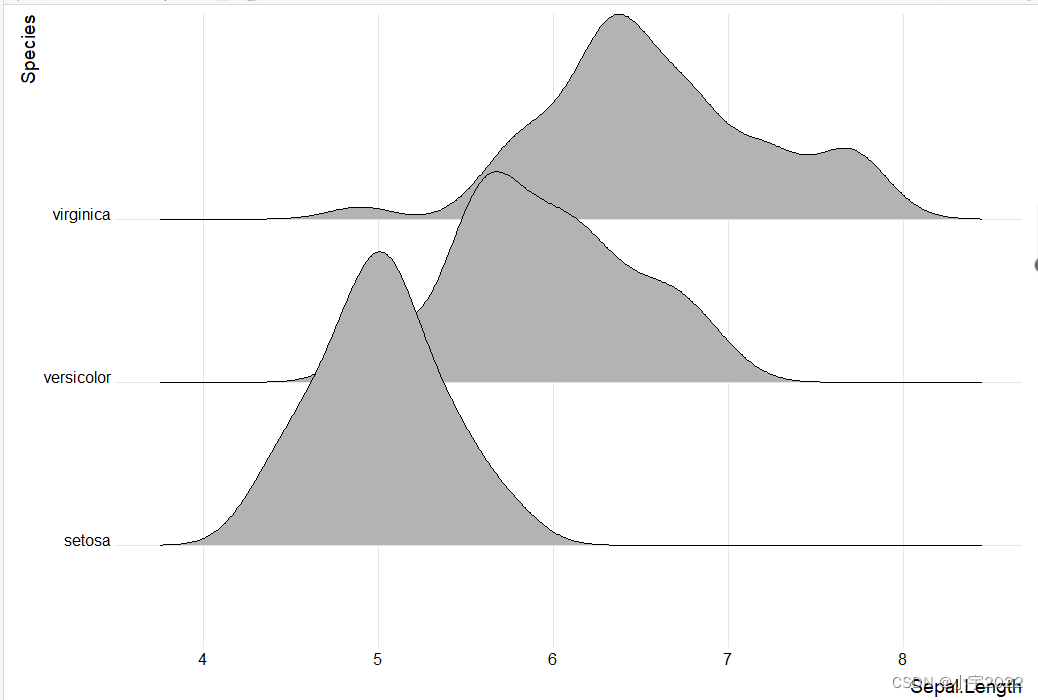``````# Remove grids
ggplot(iris, aes(x = Sepal.Length, y = Species)) +
geom_density_ridges() +
theme_ridges(grid = FALSE, center_axis_labels = TRUE)
``````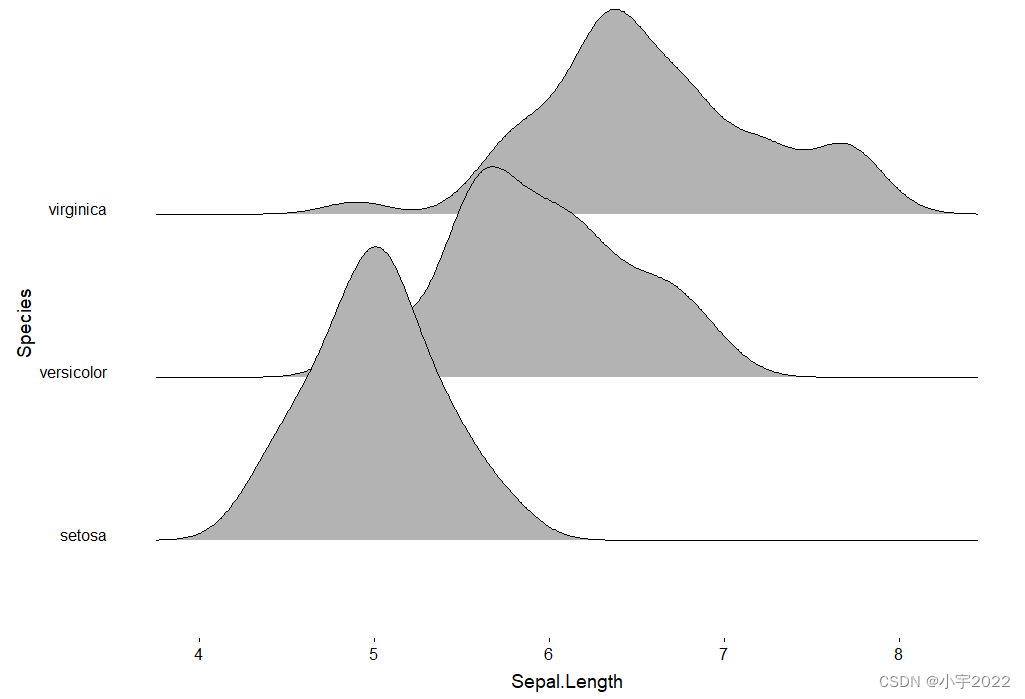https://www.datanovia.com/en/blog/elegant-visualization-of-density-distribution-in-r-using-ridgeline/### 低价透明### 金牌服务### 信息保密